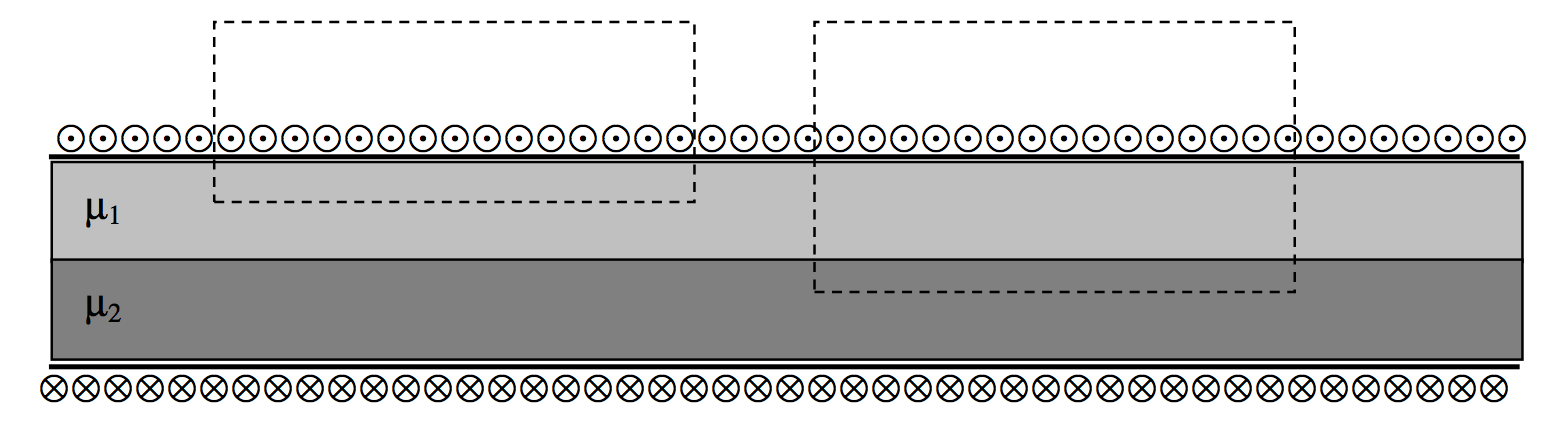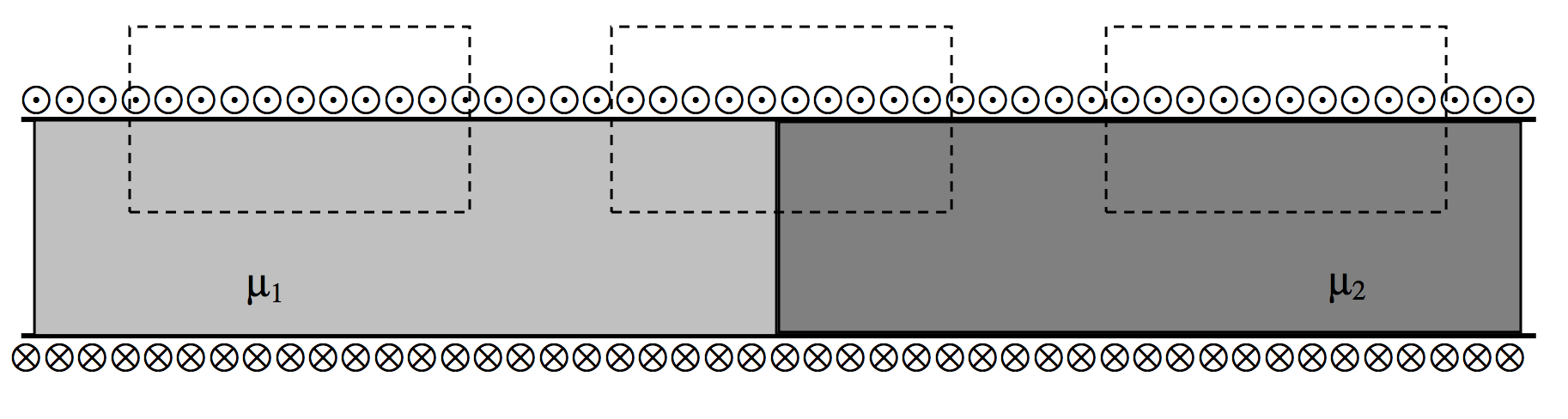$$\require{cancel}$$

# 6.12: Boundary Conditions

•• Contributed by Jeremy Tatum
• Emeritus Professor (Physics & Astronomy) at University of Victoria

We recall from Section 5.14, that, at a boundary between two media of different permittivities, the normal component of $$\textbf{D}$$ and the tangential component of $$\textbf{E}$$ are continuous, while the tangential component of $$\textbf{D}$$ is proportional to $$\epsilon$$ and the normal component of $$\textbf{E}$$ is inversely proportional to $$\epsilon$$. The lines of electric force are refracted at a boundary in such a manner that

$\frac{\tan \theta_1 }{\tan \theta_2}=\frac{\epsilon_1}{\epsilon_2}.$

The situation is similar with magnetic fields. That is, at a boundary between two media of different permeabilities, the normal component of $$\textbf{B}$$ and the tangential component of $$\textbf{H}$$ are continuous, while the tangential component of $$\textbf{B}$$is proportional to m and the normal component of $$\textbf{H}$$ is inversely proportional to $$\mu$$. The lines of magnetic force are refracted at a boundary in such a manner that

$\frac{\tan \theta_1 }{\tan \theta_2}=\frac{\mu_1}{\mu_2}.$$$\text{FIGURE VI.16}$$

The configuration of the magnetic field inside an infinitely long solenoid with materials of different permeabilities needs some care. We shall be guided by the Biot-Savart law, namely $$B=\frac{\mu I \,ds\,\sin \theta}{4\pi r}$$, and Ampère’s law, namely that the line integral of $$\textbf{H}$$ around a closed circuit is equal to the enclosed current. We also recall that the magnetic field inside an infinite solenoid containing a single homogeneous isotropic material is uniform, is parallel to the axis of the solenoid, and is given by $$H=nI$$ or $$B=\mu nI$$.

The easiest two-material case to consider is that in which the two materials are arranged in parallel as in Figure VI.17.$$\text{FIGURE VI.17}$$

One can see by applying Ampère’s law to each of the two circuits indicated by dashed lines that the $$H$$-field is the same in each material and is equal to $$nI$$, and is uniform throughout the solenoid. It is directed parallel to the axis of the solenoid. That is, the tangential component of $$\textbf{H}$$is continuous. The $$B$$-fields in the two materials, however, are different, being $$\mu_1 nI$$ in the upper material and $$\mu_2 nI$$ in the lower.

We now look at the situation in which the two materials are in series, as in Figure VI.18.

We’ll use a horizontal coordinate $$x$$, which is zero at the boundary, negative to the left of it, and positive to the right of it.$$\text{FIGURE VI.18}$$

We might at first be tempted to suppose that $$B=\mu_1 nI$$ to the left of the boundary and $$B=\mu_2 nI$$ to the right of the boundary, while, by an application of Ampère’s law around any of the dashed circuits indicated, $$H=nI$$ on both sides. Tempting though this is, it is not correct, and we shall see why shortly.

The $$B$$-field is indeed $$\mu_1 nI$$ a long way to the left of the boundary, and $$\mu_2 nI$$ a long way to the right. However, near to the boundary it is between these limiting values. We can calculate the $$B$$-field on the axis at the boundary by the same method that we used in Section 6.8. See especially equation 6.8, which, with the present geometry, becomes

$B=\frac{1}{2}\mu_1 nI \int_{-\pi/2}^{0}\cos \theta \, d\theta + \frac{1}{2}\mu_2 nI \int_{0}^{\pi /2}\cos \theta \, d\theta .\label{6.12.1}$

It should come as no surprise that this comes to

$B=\frac{1}{2}(\mu_1 + \mu_2 ) nI.\label{6.12.2}$

It is the same just to the left of the boundary and just to the right.

The $$H$$-field, however, drops suddenly at the boundary from $$\frac{1}{2}\left ( 1+\frac{\mu_2}{\mu_1}\right ) nI$$ immediately to the left of the boundary to $$\frac{1}{2}\left ( 1+\frac{\mu_1}{\mu_2}\right ) nI$$ immediately to the right of the boundary.

In any case, the very important results from these considerations is

• At a boundary between two media of different permeabilities, the parallel component of $$\textbf{H}$$ is continuous, and the perpendicular component of $$\textbf{B}$$ is continuous.

Compare and contrast this with the electrical case:

• At a boundary between two media of different permittivities, the parallel component of $$\textbf{E}$$ is continuous, and the perpendicular component of $$\textbf{D}$$ is continuous.